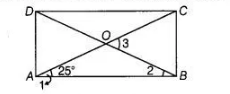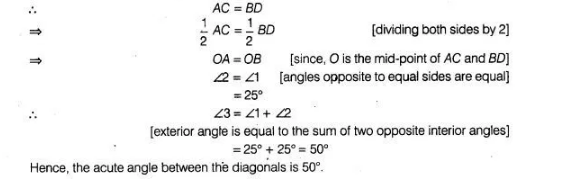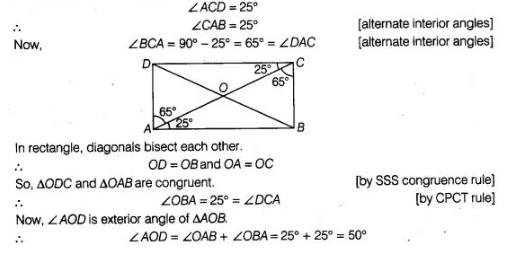# A diagonal of a rectangle is inclined to`
Question:

A diagonal of a rectangle is inclined to one side of the rectangle at $25^{\circ}$. The acute angle between the diagonals is

(a) $55^{\circ}$

(b) $50^{\circ}$

(c) $40^{\circ}$

(d) $25^{\circ}$

Solution:

(b) We know that, diagonals of a rectangle are equal in length.Alternate Method

Given, in a rectangle $A B C D$,